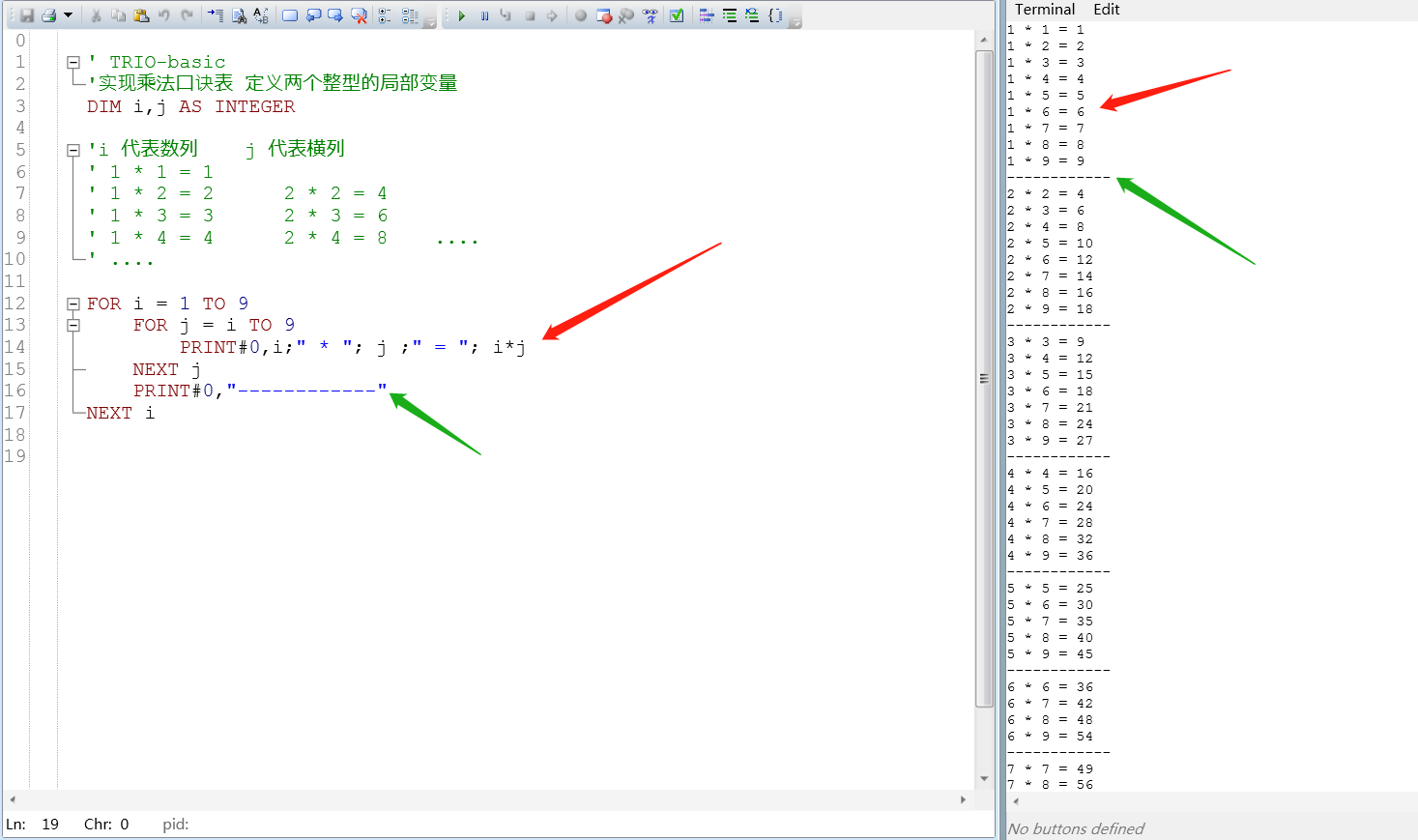# TRIO-basic指令--九九乘法表demo

2019/04/11 19:05

``````' TRIO-basic
'实现乘法口诀表 定义两个整型的局部变量
DIM i,j AS INTEGER

'i 代表数列    j 代表横列
' 1 * 1 = 1
' 1 * 2 = 2      2 * 2 = 4
' 1 * 3 = 3      2 * 3 = 6
' 1 * 4 = 4      2 * 4 = 8

FOR i = 1 TO 9
FOR j = i TO 9
PRINT#0,i;" * "; j ;" = "; i*j
NEXT j
PRINT#0,"------------"
NEXT i``````

下面是用TIOR实现的结果：0
0 收藏

### 作者的其它热门文章0 评论
0 收藏
0